# How to Round Numbers that Meet Specified Criteria in Excel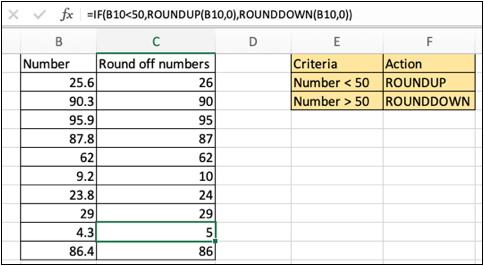In this article, we will learn How to Round Numbers that Meet Specified Criteria in Excel.

Scenario:

In Excel, working with numbers data. Sometimes we come across a problem stating rounding off numbers having met a specific criteria. For example getting round up if less than 100, round down is not. Or. Rounding up to nearest given numbers like 25, 100, 500, 1000. There are many ways to interpret conditional rounding of numbers.

1. Rounding up or down if less than or equal to a number. (48.9  -> 49, 52.9  ->  52)
2. Rounding up to nearest given whole numbers. (30,  90, 120).
3. Round up to nth digit number using ROUNDUP function.

Let's learn how to use these methods, explained below illustrating an example.

Rounding up or down if less than or equal to a number. (48.9  -> 49, 52.9  ->  52)

Here we have given a set of numbers and we need to round up or round down to the nearest integer given a criteria. Criteria is that, if the number is less than 50, then it should be rounded up and if the number is greater than or equal to 50 it should be rounded down.

Formula Syntax:

 =IF(number

number : The Number to round up

Criteria : specific number to check with

0 : num_digit (Up to digit, we need to round up or down).

Example :

All of these might be confusing to understand. Let's understand how to use the function using an example. Here In one Column there are Numbers and and one criteria table is given. Now we start rounding numbers using the formula

Use the formula

 =IF(B2<50,ROUNDUP(B2,0),ROUNDDOWN(B2,0))Explanation:

First condition is checked using the IF function. Then the number is sent to round up or round down given that the specific criteria is matched or not. If you get confuse using the IF function, Learn more about IF function here at exceltip.com

Rounding up to nearest given whole numbers. (30,  90, 120)

Here we are given a specific criteria that given number be rounded off to that particular given number. 20 => 50, 55 => 100, 433 => 500. So for that we can use nested IF function but it will be lengthier if the numbers are more. So we use a trick out of INDEX and MATCH function to round off to the nearest given number. This formula gives us the roundup functionality. Let's first get the formula syntax for the same.

Formula Syntax:

 = INDEX (list, MATCH (number, list, -1))

number : The Number to round up

List : given set of numbers, round up to

-1 : to match the last matched number

There's only one problem with this formula that you cannot match 26 -> 25, it will either go up or down. Sort the list in descending to get the rounddown functionality with the same formula

Example :

All of these might be confusing to understand. Let's understand how to use the function using an example. Here In one Column there are Numbers and and one criteria table is given. Now we start rounding numbers using the formula.

Use the formula

 =INDEX (\$A\$1:\$A\$5, MATCH (B1, \$A\$1:\$A\$5, -1))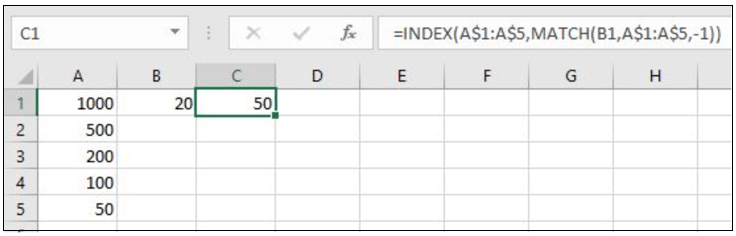Explanation:

MATCH function matches the exact minimum value match in the list (A1:A5) and returns its row index to the INDEX function.

The INDEX function finds the nearest match having ROW index and 5th row in the list

Note:

The function returns the #NA error if the lookup array argument to the MATCH function is not of the same length of the table array. Use INDEX and MATCH to Lookup Value : INDEX & MATCH function to look up value as required.

Round up to nth digit number using ROUNDUP function.

ROUNDUP function used to round up the numbers 0-4 and round up the numbers 5-9 It takes 2 arguments

Syntax:

 =ROUNDUP(number, num_digit)

number : The Number to round up

num_digit : Up to digit, we need to round up.

Example :

All of these might be confusing to understand. Let's understand how to use the function using an example. Here In one Column there are Numbers and in another, there are num_digits up to which the number to be rounded up.

Use the formula

 =ROUNDUP(A2,B2)

Here in C2 cell, the number will be round up to 1 decimal place.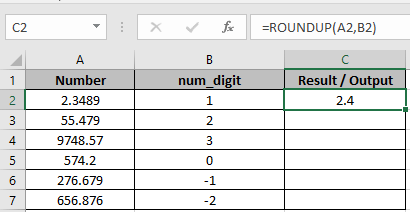As you can see 2.4 is  rounded up to 1 decimal place.

Copy the formula using Ctrl + D taking the first cell till the last cell needed formula.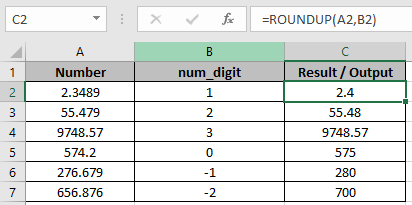As you can see

First 3 cells are rounded up upto 1, 2 and 3 decimal places respectively.

In C5, The number is rounded up to the nearest whole number.

The last 2 cells are rounded up to the nearest 10 and 100 respectively.

 To get the number rounded up to the nearest whole number, 0 is used default as num_digit

ROUND to nearest hundred or thousand

Here in range A2:A8, I have some numbers. I want to round them to 100. To do so I will write the formula below.

 =ROUND(A2,-2)

Copy this in below cells.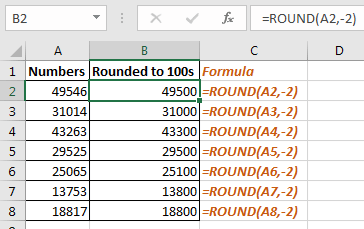How it works:

Actually when we pass a negative number to the ROUND function, it rounds the numbers before the decimal point.

So to round to nearest 1000 we just need to replace -2 to -3 in formula.

Here are all the observational notes using the formula in Excel
Notes :

1. The formula only works with numbers.
2. The formula returns the nearest last multiple given input any decimal number.
3. Negative significance is only allowed when number itself is negative else the formula returns #NUM! error.
4. Blank cell or 0 as significance value returns #DIV/0! error.
5. The function returns #VALUE! Error if the date or time value is invalid.
6. Excel stores time value as numbers. So check the format of the cell while operating this formula.
7. Careful while Input time values 04:10:22 means 4 hours, 10 minutes and 22 seconds.

Hope this article about How to Round Numbers that Meet Specified Criteria in Excel is explanatory. Find more articles on calculating values and related Excel formulas here. If you liked our blogs, share it with your friends on Facebook. And also you can follow us on Twitter and Facebook. We would love to hear from you, do let us know how we can improve, complement or innovate our work and make it better for you. Write to us at info@exceltip.com.

Related Articles :

How to use the ROUND function in Excel : Rounds off the given number to the nearest num_digit decimal using the ROUND function in Excel.

How to use the ROUNDUP function in Excel : Rounds up the given number to the nearest num_digit decimal using the ROUNDUP function in Excel.

How to use the ROUNDDOWN function in Excel : Rounds down the given number to the nearest num_digit decimal using the ROUNDDOWN function in Excel.

How to use the MROUND function in Excel : rounds off the number to the nearest specified multiple using the MROUND function in Excel.

How to use the FLOOR function in Excel : rounds down the number to the nearest specified multiple using the FLOOR function in Excel.

Popular Articles :

50 Excel Shortcuts to Increase Your Productivity : Get faster at your tasks in Excel. These shortcuts will help you increase your work efficiency in Excel.

How to use the VLOOKUP Function in Excel : This is one of the most used and popular functions of excel that is used to lookup value from different ranges and sheets.

How to use the IF Function in Excel : The IF statement in Excel checks the condition and returns a specific value if the condition is TRUE or returns another specific value if FALSE.

How to use the SUMIF Function in Excel : This is another dashboard essential function. This helps you sum up values on specific conditions.

How to use the COUNTIF Function in Excel : Count values with conditions using this amazing function. You don't need to filter your data to count specific values. Countif function is essential to prepare your dashboard.

Terms and Conditions of use

The applications/code on this site are distributed as is and without warranties or liability. In no event shall the owner of the copyrights, or the authors of the applications/code be liable for any loss of profit, any problems or any damage resulting from the use or evaluation of the applications/code.